Publicité

# Cfa corporate finance chapter3

Auditor à gisilowati
6 Sep 2017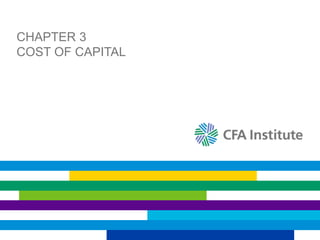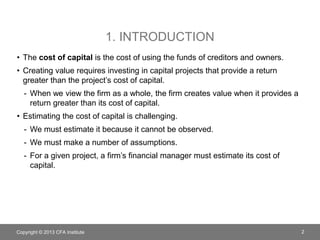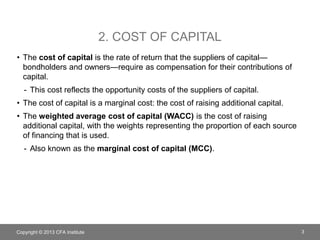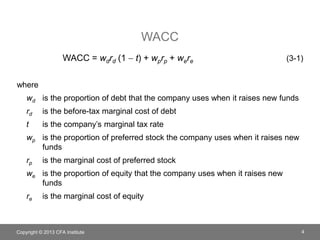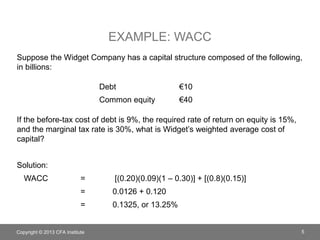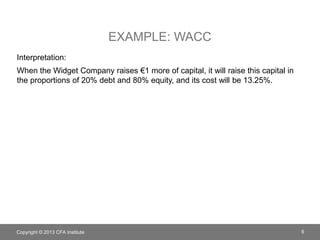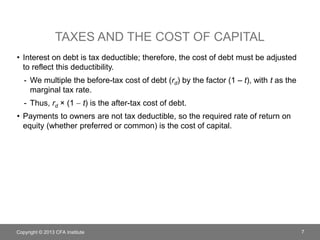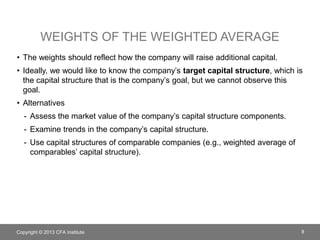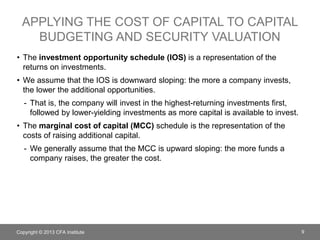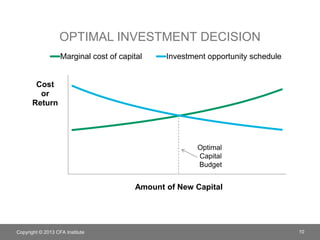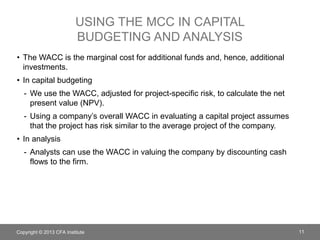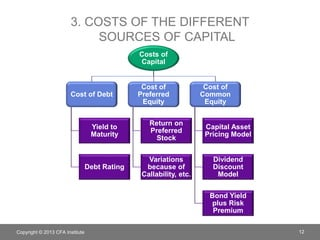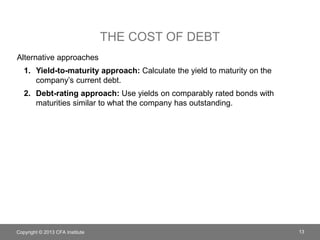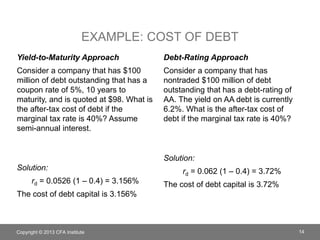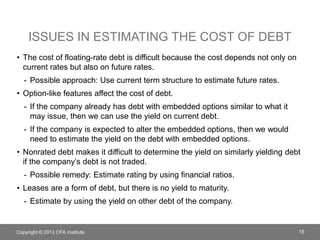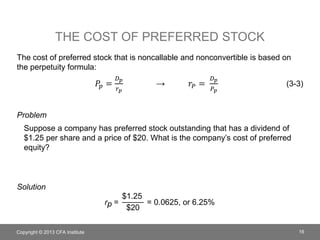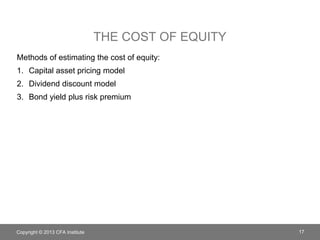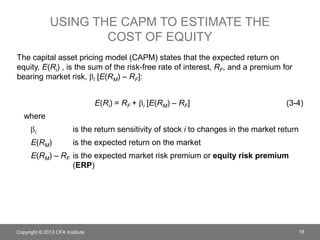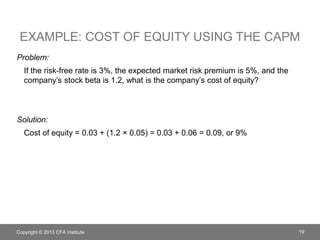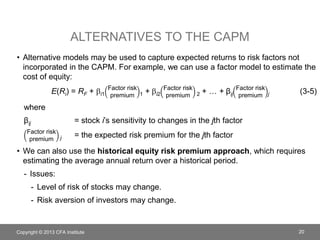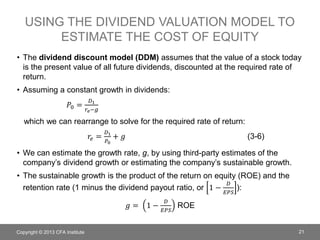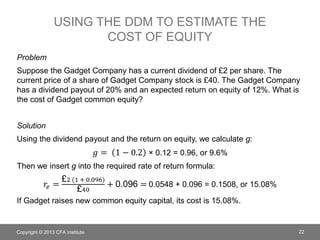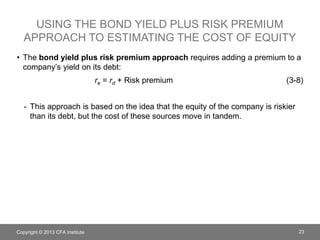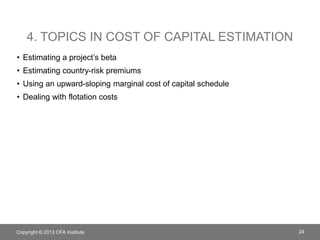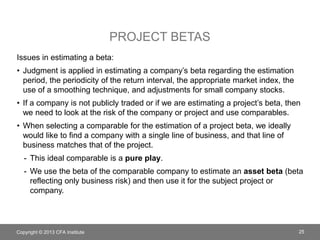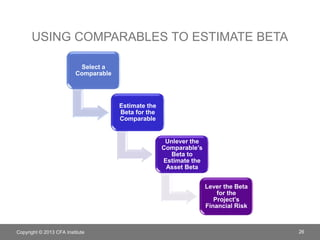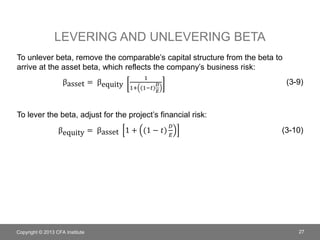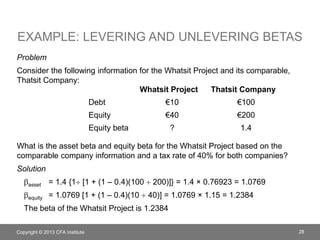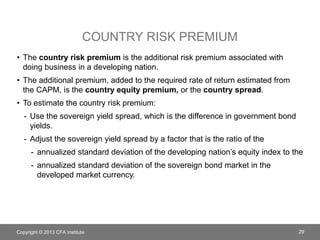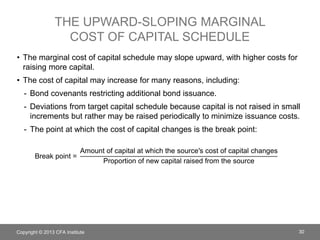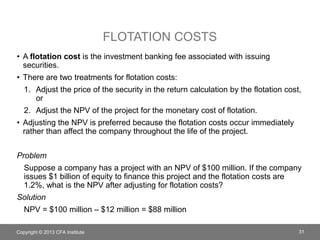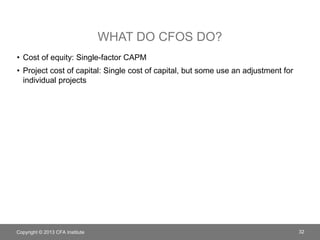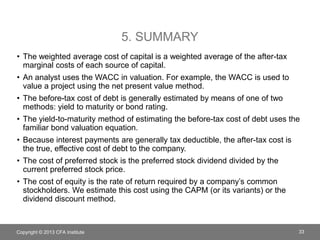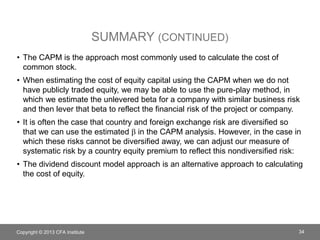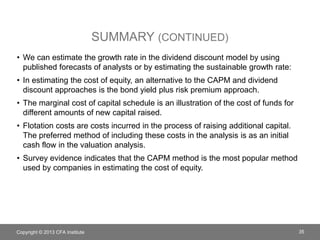1 sur 35
Publicité

### Cfa corporate finance chapter3

1. CHAPTER 3 COST OF CAPITAL
2. 1. INTRODUCTION • The cost of capital is the cost of using the funds of creditors and owners. • Creating value requires investing in capital projects that provide a return greater than the project’s cost of capital. - When we view the firm as a whole, the firm creates value when it provides a return greater than its cost of capital. • Estimating the cost of capital is challenging. - We must estimate it because it cannot be observed. - We must make a number of assumptions. - For a given project, a firm’s financial manager must estimate its cost of capital. Copyright © 2013 CFA Institute 2
3. 2. COST OF CAPITAL • The cost of capital is the rate of return that the suppliers of capital— bondholders and owners—require as compensation for their contributions of capital. - This cost reflects the opportunity costs of the suppliers of capital. • The cost of capital is a marginal cost: the cost of raising additional capital. • The weighted average cost of capital (WACC) is the cost of raising additional capital, with the weights representing the proportion of each source of financing that is used. - Also known as the marginal cost of capital (MCC). Copyright © 2013 CFA Institute 3
4. WACC WACC = wdrd (1  t) + wprp + were (3-1) where wd is the proportion of debt that the company uses when it raises new funds rd is the before-tax marginal cost of debt t is the company’s marginal tax rate wp is the proportion of preferred stock the company uses when it raises new funds rp is the marginal cost of preferred stock we is the proportion of equity that the company uses when it raises new funds re is the marginal cost of equity Copyright © 2013 CFA Institute 4
5. EXAMPLE: WACC Suppose the Widget Company has a capital structure composed of the following, in billions: If the before-tax cost of debt is 9%, the required rate of return on equity is 15%, and the marginal tax rate is 30%, what is Widget’s weighted average cost of capital? Solution: WACC = [(0.20)(0.09)(1 – 0.30)] + [(0.8)(0.15)] = 0.0126 + 0.120 = 0.1325, or 13.25% Copyright © 2013 CFA Institute 5 Debt €10 Common equity €40
6. EXAMPLE: WACC Interpretation: When the Widget Company raises €1 more of capital, it will raise this capital in the proportions of 20% debt and 80% equity, and its cost will be 13.25%. Copyright © 2013 CFA Institute 6
7. TAXES AND THE COST OF CAPITAL • Interest on debt is tax deductible; therefore, the cost of debt must be adjusted to reflect this deductibility. - We multiple the before-tax cost of debt (rd) by the factor (1 – t), with t as the marginal tax rate. - Thus, rd × (1  t) is the after-tax cost of debt. • Payments to owners are not tax deductible, so the required rate of return on equity (whether preferred or common) is the cost of capital. Copyright © 2013 CFA Institute 7
8. WEIGHTS OF THE WEIGHTED AVERAGE • The weights should reflect how the company will raise additional capital. • Ideally, we would like to know the company’s target capital structure, which is the capital structure that is the company’s goal, but we cannot observe this goal. • Alternatives - Assess the market value of the company’s capital structure components. - Examine trends in the company’s capital structure. - Use capital structures of comparable companies (e.g., weighted average of comparables’ capital structure). Copyright © 2013 CFA Institute 8
9. APPLYING THE COST OF CAPITAL TO CAPITAL BUDGETING AND SECURITY VALUATION • The investment opportunity schedule (IOS) is a representation of the returns on investments. • We assume that the IOS is downward sloping: the more a company invests, the lower the additional opportunities. - That is, the company will invest in the highest-returning investments first, followed by lower-yielding investments as more capital is available to invest. • The marginal cost of capital (MCC) schedule is the representation of the costs of raising additional capital. - We generally assume that the MCC is upward sloping: the more funds a company raises, the greater the cost. Copyright © 2013 CFA Institute 9
10. OPTIMAL INVESTMENT DECISION Copyright © 2013 CFA Institute 10 Cost or Return Amount of New Capital Marginal cost of capital Investment opportunity schedule Optimal Capital Budget
11. USING THE MCC IN CAPITAL BUDGETING AND ANALYSIS • The WACC is the marginal cost for additional funds and, hence, additional investments. • In capital budgeting - We use the WACC, adjusted for project-specific risk, to calculate the net present value (NPV). - Using a company’s overall WACC in evaluating a capital project assumes that the project has risk similar to the average project of the company. • In analysis - Analysts can use the WACC in valuing the company by discounting cash flows to the firm. Copyright © 2013 CFA Institute 11
12. 3. COSTS OF THE DIFFERENT SOURCES OF CAPITAL Costs of Capital Cost of Debt Yield to Maturity Debt Rating Cost of Preferred Equity Return on Preferred Stock Variations because of Callability, etc. Cost of Common Equity Capital Asset Pricing Model Dividend Discount Model Bond Yield plus Risk Premium Copyright © 2013 CFA Institute 12
13. THE COST OF DEBT Alternative approaches 1. Yield-to-maturity approach: Calculate the yield to maturity on the company’s current debt. 2. Debt-rating approach: Use yields on comparably rated bonds with maturities similar to what the company has outstanding. Copyright © 2013 CFA Institute 13
14. EXAMPLE: COST OF DEBT Yield-to-Maturity Approach Consider a company that has \$100 million of debt outstanding that has a coupon rate of 5%, 10 years to maturity, and is quoted at \$98. What is the after-tax cost of debt if the marginal tax rate is 40%? Assume semi-annual interest. Solution: rd = 0.0526 (1 – 0.4) = 3.156% The cost of debt capital is 3.156% Debt-Rating Approach Consider a company that has nontraded \$100 million of debt outstanding that has a debt-rating of AA. The yield on AA debt is currently 6.2%. What is the after-tax cost of debt if the marginal tax rate is 40%? Solution: rd = 0.062 (1 – 0.4) = 3.72% The cost of debt capital is 3.72% Copyright © 2013 CFA Institute 14
15. ISSUES IN ESTIMATING THE COST OF DEBT • The cost of floating-rate debt is difficult because the cost depends not only on current rates but also on future rates. - Possible approach: Use current term structure to estimate future rates. • Option-like features affect the cost of debt. - If the company already has debt with embedded options similar to what it may issue, then we can use the yield on current debt. - If the company is expected to alter the embedded options, then we would need to estimate the yield on the debt with embedded options. • Nonrated debt makes it difficult to determine the yield on similarly yielding debt if the company’s debt is not traded. - Possible remedy: Estimate rating by using financial ratios. • Leases are a form of debt, but there is no yield to maturity. - Estimate by using the yield on other debt of the company. Copyright © 2013 CFA Institute 15
16. THE COST OF PREFERRED STOCK The cost of preferred stock that is noncallable and nonconvertible is based on the perpetuity formula: 𝑃𝑝 = 𝐷 𝑝 𝑟 𝑝 → 𝑟𝑃 = 𝐷 𝑝 𝑃 𝑝 (3-3) Problem Suppose a company has preferred stock outstanding that has a dividend of \$1.25 per share and a price of \$20. What is the company’s cost of preferred equity? Solution rp = \$1.25 \$20 = 0.0625, or 6.25% Copyright © 2013 CFA Institute 16
17. THE COST OF EQUITY Methods of estimating the cost of equity: 1. Capital asset pricing model 2. Dividend discount model 3. Bond yield plus risk premium Copyright © 2013 CFA Institute 17
18. USING THE CAPM TO ESTIMATE THE COST OF EQUITY The capital asset pricing model (CAPM) states that the expected return on equity, E(Ri) , is the sum of the risk-free rate of interest, RF, and a premium for bearing market risk, bi [E(RM) – RF]: E(Ri) = RF + bi [E(RM) – RF] (3-4) where bi is the return sensitivity of stock i to changes in the market return E(RM) is the expected return on the market E(RM) – RF is the expected market risk premium or equity risk premium (ERP) Copyright © 2013 CFA Institute 18
19. EXAMPLE: COST OF EQUITY USING THE CAPM Problem: If the risk-free rate is 3%, the expected market risk premium is 5%, and the company’s stock beta is 1.2, what is the company’s cost of equity? Solution: Cost of equity = 0.03 + (1.2 × 0.05) = 0.03 + 0.06 = 0.09, or 9% Copyright © 2013 CFA Institute 19
20. ALTERNATIVES TO THE CAPM • Alternative models may be used to capture expected returns to risk factors not incorporated in the CAPM. For example, we can use a factor model to estimate the cost of equity: E(Ri) = RF + bi1 Factor risk premium 1 + bi2 Factor risk premium 2 + … + βij Factor risk premium j (3-5) where βij = stock i’s sensitivity to changes in the jth factor Factor risk premium j = the expected risk premium for the jth factor • We can also use the historical equity risk premium approach, which requires estimating the average annual return over a historical period. - Issues: - Level of risk of stocks may change. - Risk aversion of investors may change. Copyright © 2013 CFA Institute 20
21. USING THE DIVIDEND VALUATION MODEL TO ESTIMATE THE COST OF EQUITY • The dividend discount model (DDM) assumes that the value of a stock today is the present value of all future dividends, discounted at the required rate of return. • Assuming a constant growth in dividends: 𝑃0 = 𝐷1 𝑟𝑒−𝑔 which we can rearrange to solve for the required rate of return: 𝑟𝑒 = 𝐷1 𝑃0 + 𝑔 (3-6) • We can estimate the growth rate, g, by using third-party estimates of the company’s dividend growth or estimating the company’s sustainable growth. • The sustainable growth is the product of the return on equity (ROE) and the retention rate (1 minus the dividend payout ratio, or 1 − 𝐷 𝐸𝑃𝑆 ): 𝑔 = 1 − 𝐷 𝐸𝑃𝑆 ROE Copyright © 2013 CFA Institute 21
22. USING THE DDM TO ESTIMATE THE COST OF EQUITY Problem Suppose the Gadget Company has a current dividend of £2 per share. The current price of a share of Gadget Company stock is £40. The Gadget Company has a dividend payout of 20% and an expected return on equity of 12%. What is the cost of Gadget common equity? Solution Using the dividend payout and the return on equity, we calculate g: 𝑔 = 1 − 0.2 × 0.12 = 0.96, or 9.6% Then we insert g into the required rate of return formula: 𝑟𝑒 = £2 (1 + 0.096) £40 + 0.096 = 0.0548 + 0.096 = 0.1508, or 15.08% If Gadget raises new common equity capital, its cost is 15.08%. Copyright © 2013 CFA Institute 22
23. USING THE BOND YIELD PLUS RISK PREMIUM APPROACH TO ESTIMATING THE COST OF EQUITY • The bond yield plus risk premium approach requires adding a premium to a company’s yield on its debt: re = rd + Risk premium (3-8) - This approach is based on the idea that the equity of the company is riskier than its debt, but the cost of these sources move in tandem. Copyright © 2013 CFA Institute 23
24. 4. TOPICS IN COST OF CAPITAL ESTIMATION • Estimating a project’s beta • Estimating country-risk premiums • Using an upward-sloping marginal cost of capital schedule • Dealing with flotation costs Copyright © 2013 CFA Institute 24
25. PROJECT BETAS Issues in estimating a beta: • Judgment is applied in estimating a company’s beta regarding the estimation period, the periodicity of the return interval, the appropriate market index, the use of a smoothing technique, and adjustments for small company stocks. • If a company is not publicly traded or if we are estimating a project’s beta, then we need to look at the risk of the company or project and use comparables. • When selecting a comparable for the estimation of a project beta, we ideally would like to find a company with a single line of business, and that line of business matches that of the project. - This ideal comparable is a pure play. - We use the beta of the comparable company to estimate an asset beta (beta reflecting only business risk) and then use it for the subject project or company. Copyright © 2013 CFA Institute 25
26. USING COMPARABLES TO ESTIMATE BETA Select a Comparable Estimate the Beta for the Comparable Unlever the Comparable’s Beta to Estimate the Asset Beta Lever the Beta for the Project’s Financial Risk Copyright © 2013 CFA Institute 26
27. LEVERING AND UNLEVERING BETA To unlever beta, remove the comparable’s capital structure from the beta to arrive at the asset beta, which reflects the company’s business risk: βasset = βequity 1 1+ (1−𝑡) 𝐷 𝐸 (3-9) To lever the beta, adjust for the project’s financial risk: βequity = βasset 1 + (1 − 𝑡) 𝐷 𝐸 (3-10) Copyright © 2013 CFA Institute 27
28. EXAMPLE: LEVERING AND UNLEVERING BETAS Problem Consider the following information for the Whatsit Project and its comparable, Thatsit Company: What is the asset beta and equity beta for the Whatsit Project based on the comparable company information and a tax rate of 40% for both companies? Solution basset = 1.4 {1 [1 + (1 – 0.4)(100  200)]} = 1.4 × 0.76923 = 1.0769 bequity = 1.0769 [1 + (1 – 0.4)(10  40)] = 1.0769 × 1.15 = 1.2384 The beta of the Whatsit Project is 1.2384 Copyright © 2013 CFA Institute 28 Whatsit Project Thatsit Company Debt €10 €100 Equity €40 €200 Equity beta ? 1.4
30. THE UPWARD-SLOPING MARGINAL COST OF CAPITAL SCHEDULE • The marginal cost of capital schedule may slope upward, with higher costs for raising more capital. • The cost of capital may increase for many reasons, including: - Bond covenants restricting additional bond issuance. - Deviations from target capital schedule because capital is not raised in small increments but rather may be raised periodically to minimize issuance costs. - The point at which the cost of capital changes is the break point: Break point = Amount of capital at which the source′s cost of capital changes Proportion of new capital raised from the source Copyright © 2013 CFA Institute 30
31. FLOTATION COSTS • A flotation cost is the investment banking fee associated with issuing securities. • There are two treatments for flotation costs: 1. Adjust the price of the security in the return calculation by the flotation cost, or 2. Adjust the NPV of the project for the monetary cost of flotation. • Adjusting the NPV is preferred because the flotation costs occur immediately rather than affect the company throughout the life of the project. Problem Suppose a company has a project with an NPV of \$100 million. If the company issues \$1 billion of equity to finance this project and the flotation costs are 1.2%, what is the NPV after adjusting for flotation costs? Solution NPV = \$100 million – \$12 million = \$88 million Copyright © 2013 CFA Institute 31
32. WHAT DO CFOS DO? • Cost of equity: Single-factor CAPM • Project cost of capital: Single cost of capital, but some use an adjustment for individual projects Copyright © 2013 CFA Institute 32
33. 5. SUMMARY • The weighted average cost of capital is a weighted average of the after-tax marginal costs of each source of capital. • An analyst uses the WACC in valuation. For example, the WACC is used to value a project using the net present value method. • The before-tax cost of debt is generally estimated by means of one of two methods: yield to maturity or bond rating. • The yield-to-maturity method of estimating the before-tax cost of debt uses the familiar bond valuation equation. • Because interest payments are generally tax deductible, the after-tax cost is the true, effective cost of debt to the company. • The cost of preferred stock is the preferred stock dividend divided by the current preferred stock price. • The cost of equity is the rate of return required by a company’s common stockholders. We estimate this cost using the CAPM (or its variants) or the dividend discount method. Copyright © 2013 CFA Institute 33
34. SUMMARY (CONTINUED) • The CAPM is the approach most commonly used to calculate the cost of common stock. • When estimating the cost of equity capital using the CAPM when we do not have publicly traded equity, we may be able to use the pure-play method, in which we estimate the unlevered beta for a company with similar business risk and then lever that beta to reflect the financial risk of the project or company. • It is often the case that country and foreign exchange risk are diversified so that we can use the estimated b in the CAPM analysis. However, in the case in which these risks cannot be diversified away, we can adjust our measure of systematic risk by a country equity premium to reflect this nondiversified risk: • The dividend discount model approach is an alternative approach to calculating the cost of equity. Copyright © 2013 CFA Institute 34
35. SUMMARY (CONTINUED) • We can estimate the growth rate in the dividend discount model by using published forecasts of analysts or by estimating the sustainable growth rate: • In estimating the cost of equity, an alternative to the CAPM and dividend discount approaches is the bond yield plus risk premium approach. • The marginal cost of capital schedule is an illustration of the cost of funds for different amounts of new capital raised. • Flotation costs are costs incurred in the process of raising additional capital. The preferred method of including these costs in the analysis is as an initial cash flow in the valuation analysis. • Survey evidence indicates that the CAPM method is the most popular method used by companies in estimating the cost of equity. Copyright © 2013 CFA Institute 35

### Notes de l'éditeur

1. LOS: Calculate and interpret the weighted average cost of capital (WACC) of a company. Page 128 1. Introduction The cost of capital is the cost of using the funds of creditors and owners. The cost of capital for the company as a whole is often used as a basis for estimating project costs of capital. In Chapter 2, the cost of capital (also known as the required rate of return) is project specific.
2. LOS: Calculate and interpret the weighted average cost of capital (WACC) of a company. Pages 128–129 Cost of Capital The cost of capital is the rate of return that the suppliers of capital—bondholders and owners—require as compensation for their contribution of capital. The cost of capital is a marginal cost because it is the cost associated with making an investment, so everything is at the margin (that is, incremental). Discussion question: What is the reason for the cost of capital to reflect the marginal cost of capital instead of the average or embedded cost of capital?
3. LOS: Calculate and interpret the weighted average cost of capital (WACC) of a company. Page 129 WACC Note that the costs and tax rate are all on the margin.
4. LOS: Calculate and interpret the weighted average cost of capital (WACC) of a company. Pages 128–129 Example: WACC Calculations Weight of debt = €10  (€10 + €40) = 0.20 or 20% Weight of common equity = €40  (€10 + €40) = 0.80 or 80% This example is similar to Example 3-1 but without any preferred equity.
5. LOS: Calculate and interpret the weighted average cost of capital (WACC) of a company. Example: WACC
6. LOS: Describe how taxes affect the cost of capital from different capital sources. Pages 129–130 Taxes and the Cost of Capital If the concept of tax deductibility of interest is needs more explanation, consider an example: Suppose a company has earnings before interest and taxes (EBIT) of \$100, \$200 of debt with a before-tax cost of 8%, and a 25% tax rate. Then: Without tax deductibility: EBIT \$100 Taxes –25 Net income \$75 With tax deductibility: EBIT \$100 Interest –16 Earnings for taxes \$84 Taxes –21 Net income \$63 Therefore, the tax deductibility of the \$16 of interest saves \$25–\$21 = \$4 of taxes, or 25% × \$16 = \$4.
7. LOS: Explain alternative methods of calculating the weights used in the WACC, including the use of the company’s target capital structure. Pages 131–133 Weights of the Weighted Average The target capital structure is the ideal, but as analysts, we cannot observe it. The proxies include the current market value, the extrapolated trend in the company’s capital structure, and the capital structure of comparables. Example 3-3 demonstrates the market value and the comparables approach.
8. LOS: Explain how the marginal cost of capital and the investment opportunity schedule are used to determine the optimal capital budget. Page 133–135 Applying the Cost of Capital to Capital Budgeting and Security Valuation The optimal capital budget occurs when the benefits (from the IOS schedule) equal the costs (the MCC schedule).
9. LOS: Explain how the marginal cost of capital and the investment opportunity schedule are used to determine the optimal capital budget. Pages 133–135 Optimal Investment Decision The optimal investment decision is the amount of investment in which the marginal cost of capital is equal to the return on new investment. The graph is similar to Exhibit 3-1.
10. LOS: Explain the marginal cost of capital’s role in determining the net present value of a project. Pages 134–135 Using the MCC in Capital Budgeting and Analysis Capital budgeting issues: If the project has risk that is similar to that of the firm as a whole, then using the WACC in discounting project cash flows to calculate the NPV is appropriate. What if the project is not of average risk? Without adjustment, profitable projects with below-average risk would likely be rejected and unprofitable projects with higher-than-average risk may be accepted. Security valuation issues: When discounting cash flows of the entire company (e.g., free cash flows to the firm), use the WACC. When discounting equity cash flows (e.g., dividends or free cash flows to equity), use the cost of equity.
11. LOS: Calculate and interpret the cost of fixed rate debt capital using the yield-to-maturity approach and the debt-rating approach. Page 135–138 3. Costs of the Different Sources of Capital
12. LOS: Calculate and interpret the cost of fixed rate debt capital using the yield-to-maturity approach and the debt-rating approach. Pages 135–138 The Cost of Debt Yield-to-maturity approach: Calculate the yield to maturity on existing debt. Debt-rating approach: Estimate the yield based on similarly rated debt and the average yield in that debt class.
13. LOS: Calculate and interpret the cost of fixed rate debt capital using the yield-to-maturity approach and the debt-rating approach. Pages 135–138 Example: Cost of Debt Yield to maturity PMT = 2.5 N = 20 PV = 98 FV = 100 Solve for I, then multiple by 2 → YTM = 5.26% Debt-Rating approach The yield is the yield for similarly rated bonds.
14. LOS: Calculate and interpret the cost of fixed rate debt capital using the yield-to-maturity approach and the debt-rating approach. Pages 135–138 Issues in Estimating the Cost of Debt For each issue, there are possible alternatives, although each of these alternatives may be problematic. The cost of floating-rate debt is difficult because the cost depends not only on current rates, but also on future rates. Possible approach: Use current term structure to estimate future rates (that is, forward rates). Option-like features affect the cost of debt (e.g., callability, putability, convertibility). If the company already has debt with embedded options similar to what it may issue, then we can use the yield on current debt. If the company is expected to alter the embedded options, then we need to estimate the yield on the debt with embedded options. Nonrated debt makes it difficult to determine the yield on similarly yielding debt if the company’s debt is not traded. Possible remedy: Estimate rating by using financial ratios (although this method is imprecise). Leases are a form of debt, but there is no yield to maturity. Estimate by using the yield on other debt of the company (i.e., debentures).
15. LOS: Calculate and interpret the cost of noncallable, nonconvertible preferred stock. Pages 138–140 The Cost of Preferred Stock See also Examples 3-5 and 3-6. Note: The tax rate is irrelevant for the calculation of the cost of preferred stock because the dividends on preferred stock are not tax deductible by the issuer.
16. LOS: Calculate and interpret the cost of equity capital using the capital asset pricing model approach, the dividend discount approach, and the bond yield plus risk premium approach. Page 140 The Cost of Equity
17. LOS: Calculate and interpret the cost of equity capital using the capital asset pricing model approach, the dividend discount approach, and the bond yield plus risk premium approach. Pages 140–144 Using the CAPM to Estimate the Cost of Equity The CAPM requires estimating: The risk-free rate of interest The stock’s beta The market risk premium
18. LOS: Calculate and interpret the cost of equity capital using the capital asset pricing model approach, the dividend discount approach, and the bond yield plus risk premium approach. Page 140–144 Example: Cost of Equity using the CAPM This example is similar to Example 3-7.
19. LOS: Calculate and interpret the cost of equity capital using the capital asset pricing model approach, the dividend discount approach, and the bond yield plus risk premium approach. Pages 140–144 Alternatives to the CAPM The factor models may include macroeconomic factors (e.g., arbitrage pricing theory models), company-specific factors (e.g., Fama–French models), or indices in addition to the market (e.g., industry index). The historical equity premium approach requires the estimation of the mean return over a period of time. Preferred: geometric mean return Issues: The level of the stock market risk may change over time. The risk aversion of investors may change over time.
20. LOS: Calculate and interpret the cost of equity capital using the capital asset pricing model approach, the dividend discount approach, and the bond yield plus risk premium approach. Pages 144–145 Using the Dividend Valuation Model to Estimate the Cost of Equity If dividends do not grow at a constant rate, estimating the required rate of return on equity is much more challenging. We can estimate the sustainable growth using the product of the retention rate and the return on equity. Note: Some averaging over time may be appropriate because of the variability of earning and ROE for some companies.
21. LOS: Calculate and interpret the cost of equity capital using the capital asset pricing model approach, the dividend discount approach, and the bond yield plus risk premium approach. Pages 144–145 Using the DDM to Estimate the Cost of Equity The dividend payout is 20%, so the retention ratio is 80%. The product of the retention ratio and the expected return on equity is 0.8 × 0.12 = 0.096, or 9.6%. Common error, using simply £2 in the numerator instead of the correct £2 × (1 + 0.096) = £2.192.
22. LOS: Calculate and interpret the cost of equity capital using the capital asset pricing model approach, the dividend discount approach, and the bond yield plus risk premium approach. Page 145–146 Using the Bond Yield Plus Risk Premium Approach to Estimate the Cost of Equity The bond yield plus risk premium approach requires adding a premium to a company’s yield on its debt: Required rate of return on equity = Before-tax cost of debt + Risk premium The bond yield plus risk premium approach assumes that the spread between a company’s bond yield and its required rate of return is constant.
23. LOS: Calculate and interpret the beta and cost of capital for a project. LOS: Explain the country equity risk premium in the estimation of the cost of equity for a company located in a developing market. LOS: Describe the marginal cost of capital schedule, explain why it may be upward sloping with respect to additional capital, and calculate and interpret its break points. LOS: Explain and demonstrate the correct treatment of flotation costs. Pages 146–160 4. Topics in Cost of Capital Estimation Estimating a project’s beta (project versus company beta) Estimating country-risk premiums (whether or not to add a premium and, if adding, what spread to add) Using an upward-sloping marginal cost of capital schedule (when do component costs change?) Dealing with flotation costs (typical versus preferred method)
24. LOS: Calculate and interpret the beta and cost of capital for a project. Pages 146–153 Project Betas A comparable company is a company that has the same business risk as the project (or nontraded company). A pure play is a company that is publicly traded that has a single line of business. Examples: 7-11 and McDonalds.
25. LOS: Calculate and interpret the beta and cost of capital for a project. Pages 146–153 Using Comparables to Estimate Beta The process: Select comparables. Estimate the beta for comparables. Unlever the comparable’s beta to estimate the asset beta. Lever the beta for the project’s financial risk.
26. LOS: Calculate and interpret the beta and cost of capital for a project. Pages 146–153 Levering and Unlevering Beta The formulas remove leverage (Equation 3-9) and put leverage back in (Equation 3-10). Caution: Tax rates and debt/equity may differ between the project and its comparable firm (even though the formula does not subscript these to associate these with a specific firm). Discussion question: Instead of applying this method to a capital project, how would you apply this method of levering and unlevering a beta to estimate the beta of a company that is not publicly traded? Explain your steps.
27. LOS: Calculate and interpret the beta and cost of capital for a project. Pages 146–153 Example: Levering and Unlevering Betas The key to this calculation is to remove Thatsit’s financial leverage from its beta and then use this beta (the asset beta) as the base for applying Whatsit’s leverage.
28. LOS: Explain the country equity risk premium in the estimation of the cost of equity for a company located in a developing market. Pages 153–154 Country Risk Premium In the case of a project in a developing nation, we add a risk premium. We can estimate this premium using the sovereign yield spread, which we can adjust by the ratio of the developing nation’s market index volatility to the developing nation’s bond market volatility (Equation 3-13). See Example 3-12.
29. LOS: Describe the marginal cost of capital schedule, explain why it may be upward sloping with respect to additional capital, and calculate and interpret its break points. Pages 154–157 The Upward-Sloping Marginal Cost of Capital Schedule Break points occur because of limits on the issuance of a security (e.g., imposed by bond covenants) or because of the lumpiness of issuing securities (infrequent issuance to minimize investment banking costs). We can estimate a break point by comparing the capital at which a source’s cost may change with the proportion of the source in the capital structure. There may be many break points. Discussion question: Why does the MCC slope upward?
30. LOS: Explain and demonstrate the correct treatment of flotation costs. Pages 157–159 Flotation Costs Most textbooks use the first approach, which adjusts the security price for the flotation costs (see Equation 3-16 in the text). The problem is that the resulting cost of capital is used to discount cash flows of the project that occur in the future, even though the flotation costs occur immediately (time = 0 in Chapter 2). Discussion question: Why not simply subtract the flotation cost from the proceeds of the issues in calculating the cost of capital?
31. Page 160 What Do CFOs Do? The evidence is from surveys. The dividend discount model has lost favor with companies (although it was once popular).
32. 5. Summary
33. 5. Summary
34. 5. Summary
Publicité